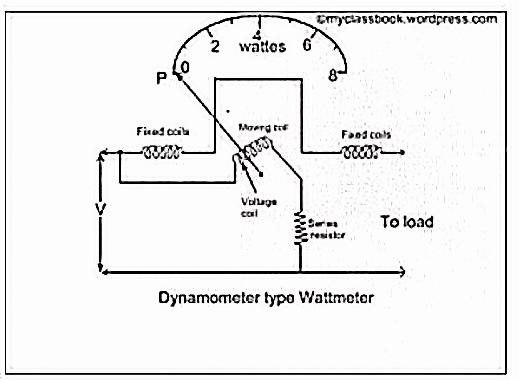### Dynamometer Type Wattmeter

It works on a very simple principle which is stated as "when any current carrying conductor is placed inside a magnetic field, it experiences a mechanical force and due to this mechanical force, deflection of conductor takes place."

Construction:
It consists of the following parts:

1. Moving coil- Moving coil moves the pointer with the help of spring control instrument. A limited amount of current flows through the moving coil so as to avoid heating. So in order to limit the current we have connected the high value resistor in series with the moving coil. The moving is air cored and is mounted on a pivoted spindle and can moves freely. In electrodynamometer type wattmeter, moving coil works as pressure coil. Hence moving coil is connected across the voltage and thus the current flowing through this coil is always proportional to the voltage.
2. Fixed coil- The fixed coil is divided into two equal parts and these are connected in series with the load, therefore the load current will flow through these coils. Now the reason is very obvious by using two fixed coils instead of one, so that it can be constructed to carry a considerable amount of electric current. These coils are called the current coils of electrodynamometer type wattmeter. Earlier these fixed coils were designed to carry the current of about 100 amperes but now the modern wattmeter are designed to carry current of about 20 amperes in order to save power.
3. Control system- Out of two controlling systems i.e. gravity control and spring control, only spring controlled systems are used in these types of wattmeter. Gravity controlled system cannot be employed because they will contain an appreciable amount of errors.
4. Damping system- Air friction damping is used, as eddy current damping will distort the weak operating magnetic field and thus it may lead to error.
5. Scale- There is a uniform scale used in these types of instrument as a moving coil moves linearly over a range of 40 degrees to 50 degrees on either side.

## When power is to be measured in a circuit, the instrument is suitably connected in the circuit. The current coil is connected in series with load so that it carries the circuit current. The potential coil is connected across the load so that it carries current proportional to the voltage. Due to the current in the coils, mechanical force exists between them. The result is that the moving coil moves the pointer over the scale. The pointer comes to rest at a position where deflecting torque is equal to the controlling torque. Reversing the current, reverses the field due to the fixed coil as well as the current in the moving coil so that the direction of the deflection torque remains unchanged. Therefore, such instruments can be used for the measurement of a.c as well as d.c power.Merits:

• Scale is uniform up to a certain limit.

• They can be used for both to measure ac as well dc quantities as scale is calibrated for both. Errors/Demerits :

• Errors in the pressure coil inductance

• Errors may be due to pressure coil capacitance

• Errors may be due to mutual inductance effects

• Errors may be due connections (i.e. pressure coil is connected after current coil)

• Error due to Eddy currents

## Pressure coil inductance

In an ideal dynamo-meter type wattmeter the current in the pressure coil is in phase with the applied voltage. But practically the pressure coil of wattmeter has an inductance and current in it will lag behind the applied voltage. If there is no inductance the current in pressure coil will be in phase with the applied voltage. In the absence of inductance in pressure coil of wattmeter, it will read correctly in all power factors and frequency. The wattmeter will read high when the load power factor is lagging ,as in that case the effect of pressure coil inductance is to reduce the phase angle between load current and pressure coil current . Hence the wattmeter will read high. This is very serious error. The wattmeter will read low when the load power factor is leading as in that case the effect of pressure coil inductance is to increase the phase angle between load current and pressure coil current. Hence the wattmeter will read low. Compensation for inductance of pressure coil. Inductance of pressure coil can be reduced by means of capacitor connected in parallel with a portion of multiplier (series resistance).